# Differential inclusion

(diff) ← Older revision | Latest revision (diff) | Newer revision → (diff)

multi-valued differential equation, differential equation with multi-valued right-hand side

A relation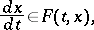(1)

where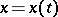is an unknown vector function on some interval and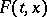is a set in an-dimensional space which depends on the numberand on the vector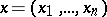. The solution of a differential inclusion (1) is usually understood to mean an absolutely-continuous vector function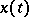which satisfies the relation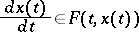almost-everywhere on the interval of variation ofunder consideration. In particular, if the setconsists of a single point, a differential inclusion becomes an ordinary differential equation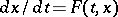. Equations of the type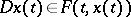where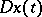is a contingent, , are equivalent to differential inclusions in a large number of cases.

Differential inclusions are generated, for example, by the problem concerning functions which satisfy a differential equation to within required accuracyby differential inequalities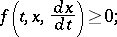by differential equations with discontinuous right-hand side , Chapt. 2; and by problems in the theory of optimal control , . The equation which is most often considered in control problems is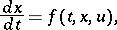(2)

whereis the vector function sought, whileis the control, i.e. a vector function which may be arbitrarily chosen out of all permissible controls (i.e. such that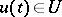for each, whereis a given set which may depend onand on). The set of solutions of equation (2) for all permissible controls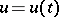satisfies the differential inclusion (1), whereis the set of all values of the functionwhenruns through the set.

In the theory of differential inclusions it is usually assumed that for any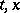from the domainunder consideration the setis a non-empty closed bounded set in an-dimensional space. If the setis everywhere convex, and, for any, it is an upper semi-continuous function in(i.e. for anyand anythe set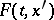is contained in the-neighbourhood of the setfor all sufficiently small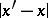), while for anyit is a measurable function of(i.e. for anyand any spherein the-dimensional space, the set of values offor which the set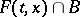is non-empty is Lebesgue measurable), and if alsois always contained in a sphere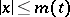where the function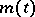is Lebesgue integrable, then, for any initial conditions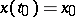,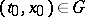, a solution of the differential inclusion exists  and the integral funnel consisting of such solutions displays the usual properties . The requirement that the setbe convex may be dropped if it depends continuously on. The existence of a solution is preserved , but the properties of the integral funnels are not.

For a review of the publications on differential inclusions and on the connection of such inclusions with control problems see , . For the concept of stability of differential inclusions see , ; for the existence of bounded and periodic solutions, and for other properties, see , , .

How to Cite This Entry:
Differential inclusion. Encyclopedia of Mathematics. URL: http://encyclopediaofmath.org/index.php?title=Differential_inclusion&oldid=13723
This article was adapted from an original article by A.F. Filippov (originator), which appeared in Encyclopedia of Mathematics - ISBN 1402006098. See original article